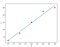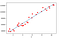Normal Equation Using Python

Necessary Imports

import numpy as np
import matplotlib.pyplot as plt
import pandas as pd

Reading and Plotting the Data

x = np.array([1,2,3,4,5])
y = np.array([7,9,12,15,16])
x = dataset.iloc[:, 0].values #Feature matrix
y = dataset.iloc[:, 1].values #Criterion Matrix

Understanding Normal Equation

ŷͥ = Ɵ˳ + Ɵࢭx
# Ɵ˳ is the intercept and Ɵࢭ is the slope of the line.

Python Code

x _ bias = np.ones((m,1)) #m is number of records in the dataset.
#shape of x can be calculated by
print(x.shape) #which turns out to be (5,) for our sample dataset.
#We need to convert it to (5,1) for successful
#addition as np.ones yields us with an array
#of (5,1) dimension. Click here to know more.
x = np.reshape(x,(m,1))
updated_x = np.append(x_bias,x,axis=1) #axis=1 to join matrix using
#column.
x_transpose = np.transpose(x)   #calculating transpose
x_transpose_dot_x = x_transpose.dot(x) # calculating dot product
temp_1 = np.linalg.inv(x_transpose_dot_x) #calculating inverse
temp_2 = x_transpose.dot(y)
Ɵ = temp_1.dot(temp_2)Fitting Regression line to our Sample DatasetRegression Line for our Salary VS Exp. Dataset
import numpy as np
import matplotlib.pyplot as plt
import pandas as pd
# x = np.array([1,2,3,4,5]) #Uncomment this when using Sample Dataset# y = np.array([7,9,12,15,16]) #Uncomment this when using Sample Dataset# dataset = pd.read_csv('Salary_Data.csv') #Uncomment this when using SalaryVsExp Dataset# x = dataset.iloc[:, 0].values #Uncomment this when using SalaryVsExp Dataset# y = dataset.iloc[:, 1].values #Uncomment this when using SalaryVsExp Datasetplt.scatter(x,y,color='red')x_bias = np.ones((5,1))x = np.reshape(x,(5,1))x = np.append(x_bias,x,axis=1)x_transpose = np.transpose(x)x_transpose_dot_x = x_transpose.dot(x)temp_1 = np.linalg.inv(x_transpose_dot_x)temp_2=x_transpose.dot(y)theta =temp_1.dot(temp_2)print(theta)# y = 4.6 + 2.4*x #Uncomment this when using Sample Dataset# y = 25792.2 + 9449.96*x #Uncomment this when using SalaryVsExp Datasetplt.plot(x,y,color='blue')plt.show()
Welcome to a place where words matter. On Medium, smart voices and original ideas take center stage - with no ads in sight. Watch
Follow all the topics you care about, and we’ll deliver the best stories for you to your homepage and inbox. Explore
Get unlimited access to the best stories on Medium — and support writers while you’re at it. Just \$5/month. Upgrade